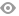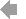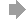﻿ Page 9 - Junior Explorer 4 - Zeszyt ćwiczeń
Basic HTML VersionView Full Version
Page 9 - Junior Explorer 4 - Zeszyt ćwiczeń
P. 9

``````Days, months and seasons

5   Odszukaj w diagramie i zapisz nazwy dni tygodnia, miesięcy i pór roku.

D   O  A   U   G  U    S  T   O   T   E  Q   N  R
E   K  U   M   E   P  A  U   E   V  J  O   F  O
C   P  T   U   N   S  D  E     B   S  A  U   S  A
E   S  U   Y  A   O  E  S     M  O  N  D  A  Y
M   A  M   O  A   R  X  D      A   L  U  C    T   B
B   L  N   L   P   B   Z  A   Y   K  A  E    U   C
E   A  N    F   R   E   O  Y    L   S  R   B  R   V
R  V   L   W   I   N   T  E   R   P  Y   E  D   O
E   N  O   F    L  W  O  A     B   L   V   S  A  P
T   O   C   T   O   B   E  R   P   J   U  L   Y   A
S  V   B     D   E  G   F   J   K  O  E   J   K   N
E  E  Q   S    U  V    A  X   L   S   B  F   A  W
P  M  A    R   C  H    E  F   L   U   P  E   S  E
T  B   R   N   K   S   P  R   I   N  G   B  O  D
E  E   J   F   A  U    V  I   A  D    P  R   S  N
M   R   A   G   K  M    K  D   L   A   E  U   P   E                  Days of the week
B  K   P   H   A  M    I  A   V  Y    P  A  M  S
E  S   J   U   N   E   K  Y   A  O  E  R     Z  D                       Monday
R  L   M   A   K   R   D  M   J   N   G  Y   F   A
U   V   S   P   Q   O   T  H   U   R   S  D   A   Y

Months
January

Seasons
spring

7``````4   5   6   7   8   9   10   11   12   13   14